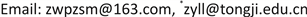1. 引言

2. 研究方法2.1. 泊松分布与极值分布

F n ( y ) = p n ( 0 ) = { λ n ( y ) } 0 0 ! e − λ n ( y ) (1)

λ n ( y ) = { − ( 1 + ξ y − μ n σ n ) − 1 ξ ξ ≠ 0 exp ( − y − μ n σ n ) ξ = 0 (2)

H u ( y ) = λ ( y , θ N ) λ ( u , θ N ) = λ ( y , θ 1 ) λ ( u , θ 1 ) y > u (3)

2.2. Delta估计

ϕ ^ ∼ N ( ϕ , V ϕ ) (4)

V ϕ = ∇ ϕ T V ( μ , σ , ξ ) ∇ ϕ (5)

∇ ϕ = [ ∂ ϕ ∂ μ , ∂ ϕ ∂ σ , ∂ ϕ ∂ ξ ] T (6)

V ( μ , σ , ξ ) 为 ( μ , σ , ξ ) 的方差协方差矩阵，计算时均以估计值代入，此方法即为delta法。

2.3. 季度模型

Z t ∼ GEV ( μ ( t ) , σ ( t ) , ξ ( t ) ) (7)

Z t ∼ GEV ( μ ( t ) , σ , ξ ) (8)

2.4. 似然比检验

2.5. 模型检测

Z t ˜ = 1 ξ ^ ( t ) log { 1 + ξ ^ ( t ) ( Z t − μ ^ ( t ) σ ^ ( t ) ) } (9)

{ i / ( m + 1 ) , exp ( − exp ( − z ˜ ( i ) ) ) ; i = 1 , ⋯ , m } (10)

{ z ˜ ( i ) , − log ( − log ( i / ( m + 1 ) ) ) ; i = 1 , ⋯ , m } (11)

2.6. 经验度试算

K = E 2 ( λ ) V ( λ ) (12)

3. 实例应用3.1. 模型拟合

μ 春 = μ 冬 (9)

μ 夏 = μ 秋 (10)

Estimation of seasonal model parameter

5.82 (0.62)6.78 (0.58)0.14 (0.06)
11.8 (0.63)6.78 (0.58)0 (0.08)
13.87 (1.15)6.78 (0.58)0.14 (0.06)
5.82 (0.62)6.78 (0.58)0.14 (0.06)

2 ( − 1227.37 − ( − 1273.54 ) ) > q c h i s q ( 0.95 , 2 ) (11)

3.2. 经验度计算

4. 结论